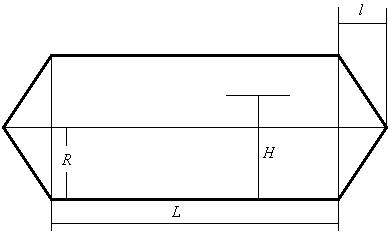# Volume-calculating function in Mathematica

## Homework Statement

Create a function MyContainer[R, L, l, H] in the program 'Mathematica' to determine the capacity of fluid in the container according to the given filling height H. The container is a cyclinder with cones on both sides.

The arguments of the function MyContainer[R, L, l, H] have to be changeable. The function has to have the property of being able to calculate the volume, irrespective of the values of the linear dimensions ( R,L,l).

Create a graph that depicts the filling height (H) dependance of the filling volume (v).I really appreciate the help, I need to get started with this assignment, but I am not sure how. I am not very crafty at using Mathematica, it is one of the course homeworks that everybody has to submit.

Great thanks!

## The Attempt at a Solution

All I know is that I have to integrate to find the volumes, but I don't know how to write down the equations and the limits of the integrals.

#### Attachments

Last edited:

Firstly, I tried to calculate the volumes of the cones, the cylinder volume is easy (no integration needed?).

I tried the following expression:

Integrate[2 [l/R] Sqrt[x^2 + z^2], {x, 0, R}, {z, -Sqrt[R^2 - x^2], Sqrt[R^2 - x^2]}, {y, Sqrt[x^2 + z^2], l}, Reals]
Simplify[%]

But unfortunately, Mathematica doesn't give any sensible result for this integral. The professor saw this effort and promised to show his own solution, hopefully I will get it solved, eventually.

Last edited:
Okay, I think I got it already, the cone stuff is relatively easy. It was all about the limits of the integrals, I needed no more specific integrand than dzdydx. Great.

The graph still needs to be created. Not difficult probably.